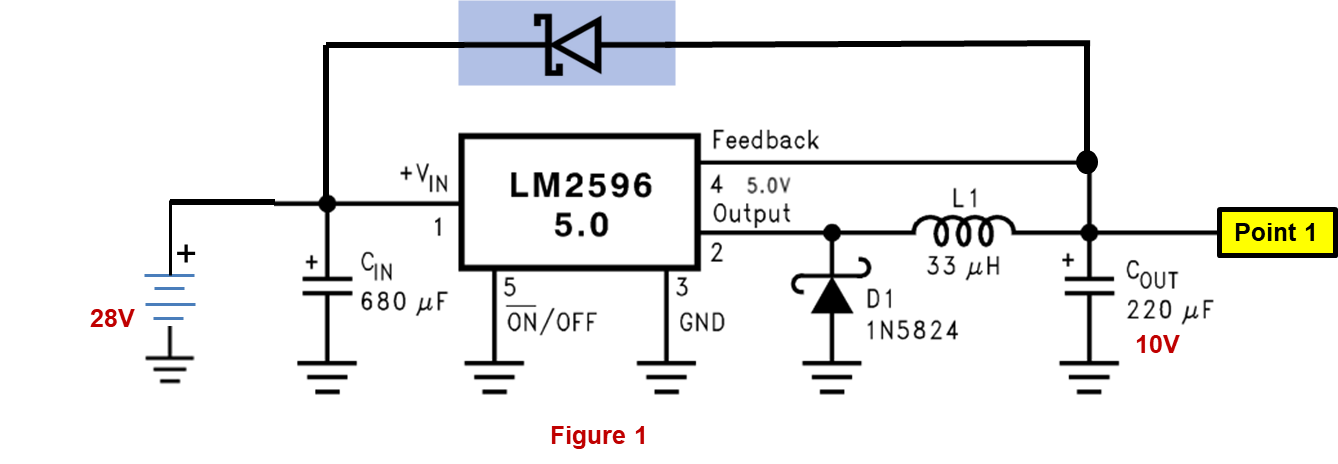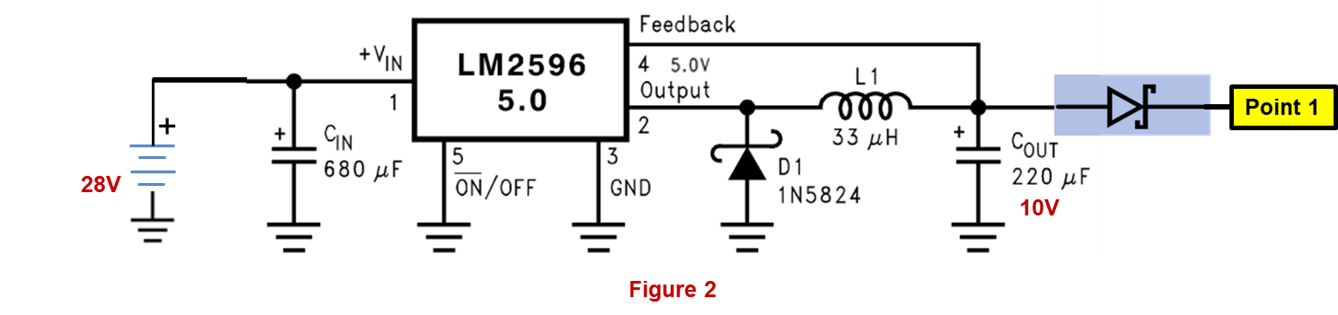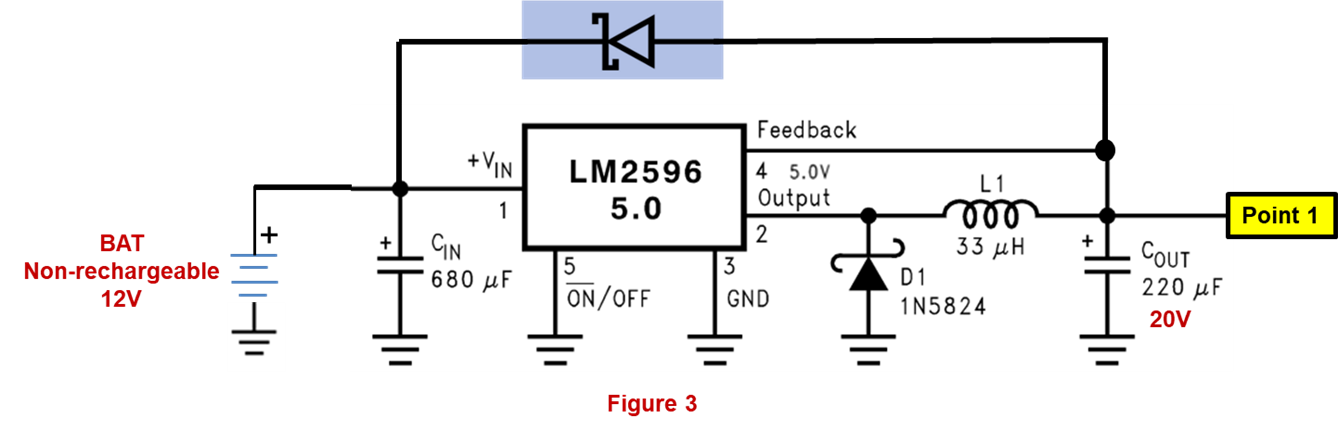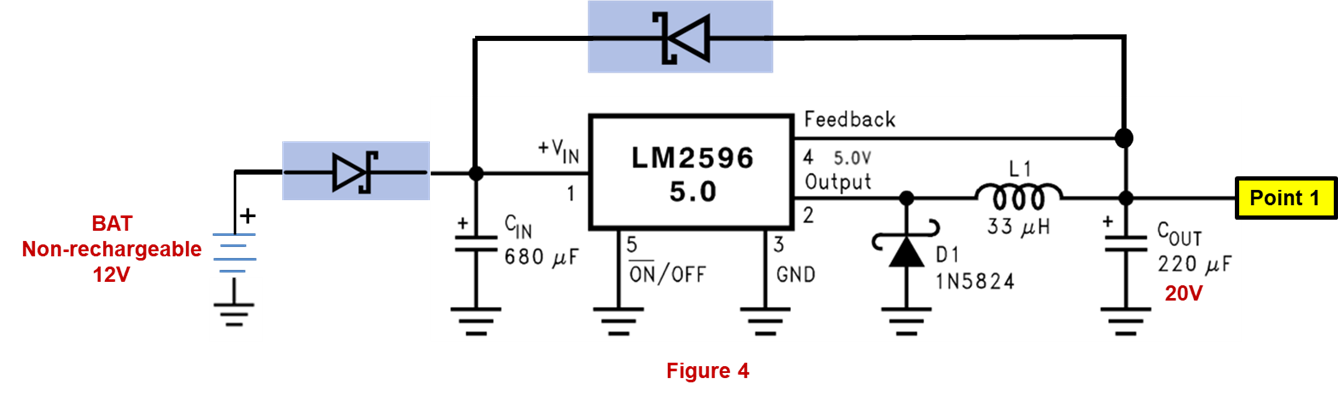If you have a related question, please click the "Ask a related question" button in the top right corner. The newly created question will be automatically linked to this question.

# LM2596: Reverse current protection of LM2596(5V out)

Part Number: LM2596

Hi all, I want to ask some questions about Reverse current protection of LM2596(5V out):

1) With Figure 1: I see that Absolute maximum ratings of Pin4 (Feedback pin) is -0.3to25V. When LM2596 is operating if I apply 28V to Point1 by mistake, Are LM2596 and Cout (Rate voltage=10V) damaged ?2) With Figure 2: when LM2596 is operating if I apply 28V to Point1 by mistake, Are LM2596 and Cout (Rate voltage=10V) damaged ? If this solution is ok, Have any disadvantages with this solution ?3) Have any application notes or technical documents about Reverse current protection of LM2596 ?

• Hello

In figure 1, the 28V is beyond the rating of pin 4 (25V).  Therefore damage will occur.

In figure 2, the device and output capacitor will be protected.  The voltage to your load will be reduced by the voltage drop of the diode.

Thanks

I would like to ask a few more questions.

In Figure 3, When LM2596 is operating if I apply 16V to Point1 by mistake,Question_4) In figure 3, Is BAT (non-rechargeable) damaged ?Question_5) If the BAT(non-rechargeable) is damaged in figure 3, Is the solution in figure 4 ok ?

• Hello

You may have your figure numbers mixed up.  I think that figure 5 is good.

However, in figure 3 the diode will also block any voltage going to the battery.

Thanks

• Sorry for the unclear between figure numbers and question numbers. So, I have edited it.

You said: "in figure 3 the diode will also block any voltage going to the battery" . But I think that in figure 3, 16V(at Point 1) will forward bias the diode so that the current will return to the non-rechargeable BAT and non-rechargeable BAT will damaged.

Am I correct in thinking so?

Regards !

• Hello

Yes; you are correct

Thanks